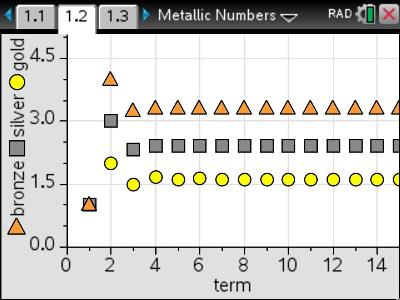# Activities

•• ##### AuthorAust Senior

30 Minutes

• ##### Device
•TI-Nspire™ CX CAS
•TI-Nspire™ CX
• ##### Software

TI-Nspire™
TI-Nspire™ CAS

4.5

## Metallic Numbers#### Activity Overview

The Golden Ratio and its association with the Fibonacci sequence is well known, but there is much more to explore. Variants of the Fibonacci sequence of the form t(n+2)=t(n)+a.t(n+1) also generate specific ratios. For a = 2 the ratio is referred to as the Silver Ratio, for a = 3 the bronze ratio, and in general, the metallic ratios. This activity explores these different ratios and compares the properties to the case where a = 1, the Golden Ratio.

#### Objectives

• Use recursion to generate a sequence
• Solve problems involving sequences
• Explore the most popular second order linear recursive relation

#### Vocabulary

• Recursion
• Fibonacci Sequence
• Golden Ratio
• Silver Ratio
• Bronze Ratio
• Metallic Ratios